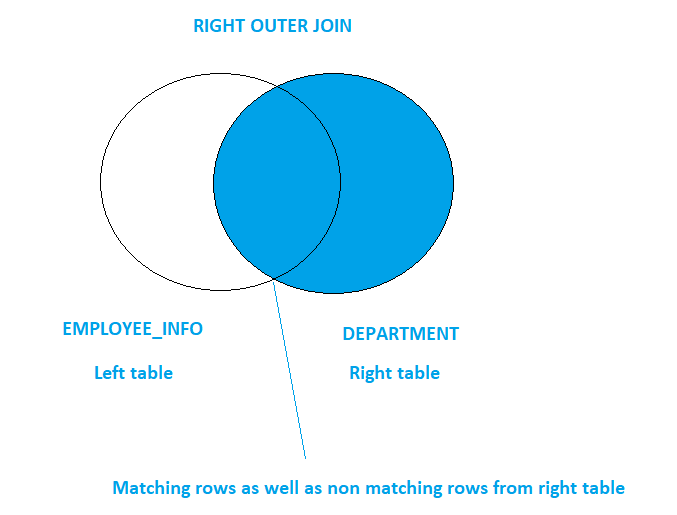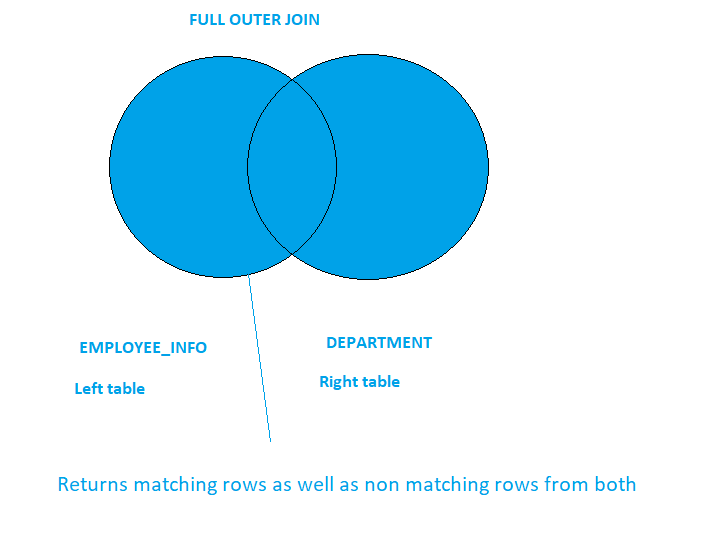Home » SQL Server JOINS

# SQL Server JOINS### A JOIN clause is used to combine two or more related tables. Tables are related to each other using foreign key constraints

There are five types of joins in SQL Server as given below

INNER JOIN – SQL Inner Join returns the rows from the tables that have matching value in columns.(inner join)

SYNTAX

``` SELECT COLUMN(S),...
FROM TABLE T1 INNER JOIN TABLE T2
ON T1.COLUMN = T2. COLUMN
WHERE CONDITIONS..```LEFT OUTER JOIN – SQL LEFT JOIN or LEFT OUTER JOIN returns all rows from the left table and the matching rows from  the  right table. If a row in the left table does not have any matching row in the right table then columns of the right table will have nulls value.(Left Outer Join)

SYNTAX

``` SELECT COLUMN(S),... FROM TABLE T1 LEFT OUTER JOIN TABLE T2
ON T1.COLUMN = T2. COLUMN
WHERE CONDITIONS..```RIGHT OUTER JOIN – SQL RIGHT JOIN or RIGHT OUTER JOIN returns all rows from the right table and the matching rows  from the left table.  If a row in the right table does not have any matching row in the left table then columns of the left table will have nulls value.(Right Outer Join)

SYNTAX

```SELECT COLUMN(S),...
FROM TABLE T1 RIGHT OUTER JOIN TABLE T2
ON T1.COLUMN = T2. COLUMN
WHERE CONDITIONS..```Read.. RIGHT OUTER JOIN WITH EXAMPLE

FULL OUTER JOIN – SQL FULL JOIN or  FULL OUTER JOIN returns rows from both left and right tables, with the matching rows from both tables where available. If there is no match, then missing side will have null values.(Full Outer Join)

SYNTAX

```SELECT COLUMN(S),...
FROM TABLE T1 FULL OUTER JOIN TABLE T2
ON T1.COLUMN = T2. COLUMN
WHERE CONDITIONS..```Read .. FULL OUTER JOIN WITH EXAMPLE

CROSS JOIN – SQL CROSS JOIN returns a result set which is the number of rows in the first table multiplied by the number of rows in the second table if no WHERE clause is used along with CROSS JOIN or we can say it returns the Cartesian product of rows from the row sets in the join. (Cross Join)

SYNTAX

`SELECT * FROM TABLE T1 CROSS JOIN TABLE T2`You can also read CROSS JOIN WITH EXAMPLE

This site uses Akismet to reduce spam. Learn how your comment data is processed.